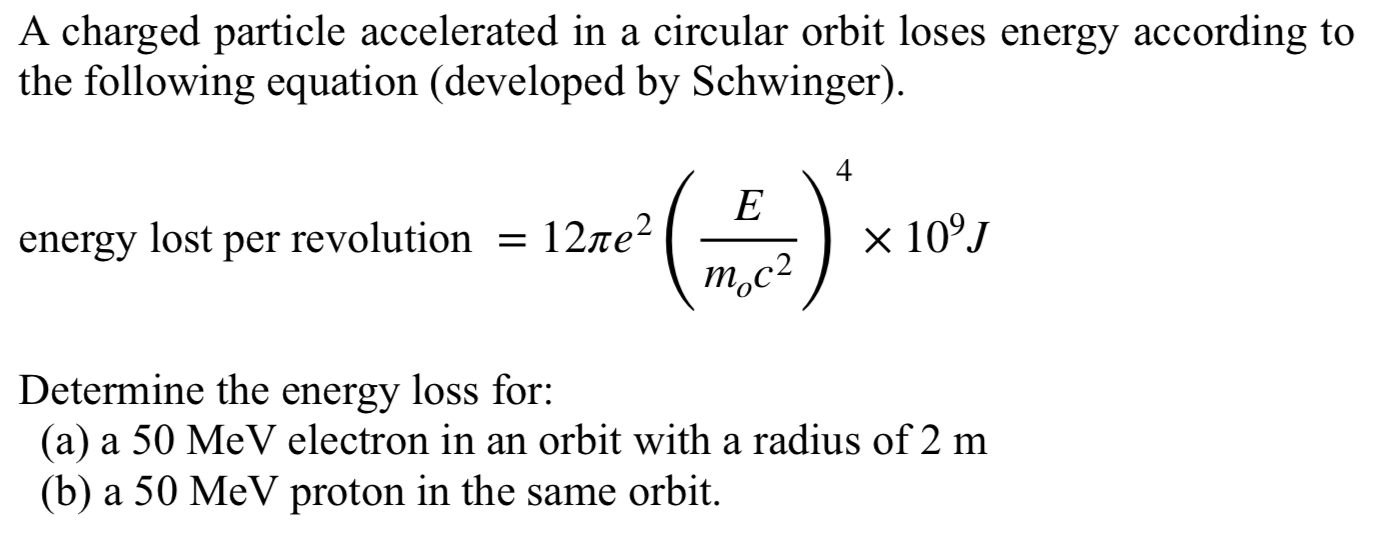Home / Expert Answers / Physics / a-charged-particle-accelerated-in-a-circular-orbit-loses-energy-according-to-the-following-equatio-pa572

# (Solved): A charged particle accelerated in a circular orbit loses energy according to the following equatio ...A charged particle accelerated in a circular orbit loses energy according to the following equation (developed by Schwinger). energy lost per revolution $$=12 \pi e^{2}\left(\frac{E}{m_{o} c^{2}}\right)^{4} \times 10^{9} \mathrm{~J}$$ Determine the energy loss for: (a) a $$50 \mathrm{MeV}$$ electron in an orbit with a radius of $$2 \mathrm{~m}$$ (b) a $$50 \mathrm{MeV}$$ proton in the same orbit.

We have an Answer from Expert